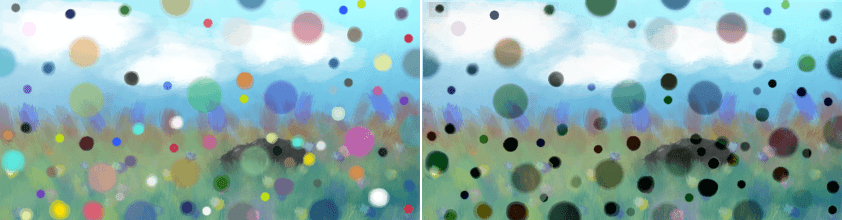# Darken¶

## Burn¶

A variation on Divide, sometimes called ‘Color Burn’ in some programs.

This inverts the bottom layer, then divides it by the top layer, and inverts the result. This results in a darkened effect that takes the colors of the lower layer into account, similar to the burn technique used in traditional darkroom photography.

1_{[1_Darker Gray(0.4, 0.4, 0.4)] / Lighter Gray(0.5, 0.5, 0.5)} = (-0.2, -0.2, -0.2) → Black(0, 0, 0)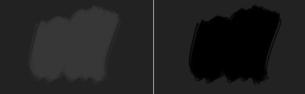Left: Normal. Right: Burn.

1_{[1_Light Blue(0.1608, 0.6274, 0.8274)] / Orange(1, 0.5961, 0.0706)} = (0.1608, 0.3749, -1.4448) → Green(0.1608, 0.3749, 0)Left: Normal. Right: Burn.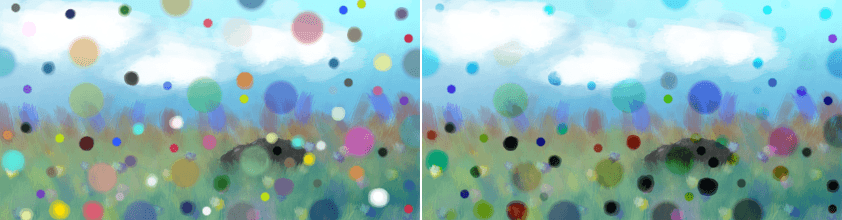Left: Normal. Right: Burn.

## Easy Burn¶

Aims to solve issues with Color Burn blending mode by using a formula which falloff is similar to Dodge, but the falloff rate is softer. It is within the range of 0.0f and 1.0f unlike Color Burn mode.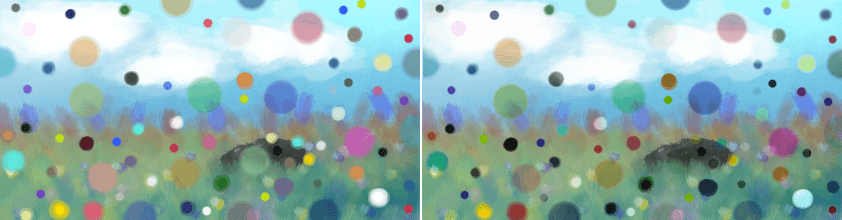Left: Normal. Right: Easy Burn.

## Fog Darken (IFS Illusions)¶

Darken the image in a way that there is a ‘fog’ in the end result. This is due to the unique property of fog darken in which midtones combined are lighter than non-midtones blend.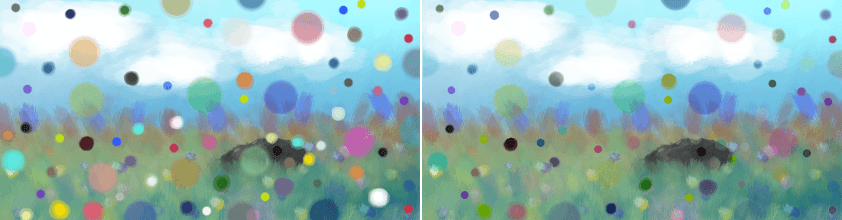Left: Normal. Right: Fog Darken (exactly the same as Addition).

## Darken¶

With the darken, the upper layer’s colors are checked for their lightness. Only if they are darker than the underlying color on the lower layer, will they be visible.

Is Lighter Gray(0.5, 0.5, 0.5) darker than Darker Gray(0.4, 0.4, 0.4)? = (no, no, no) → Darker Gray(0.4, 0.4, 0.4)Left: Normal. Right: Darken.

Is Orange(1, 0.5961, 0.0706) darker than Light Blue(0.1608, 0.6274, 0.8274)? = (no, yes, yes) → Green(0.1608, 0.5961, 0.0706)Left: Normal. Right: Darken.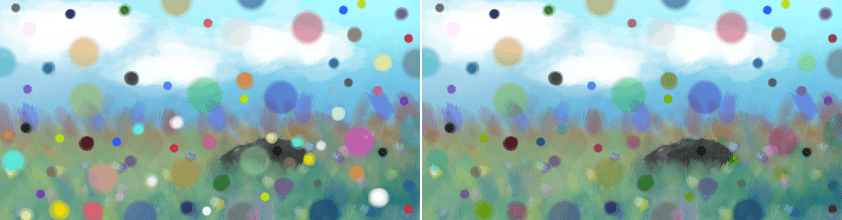Left: Normal. Right: Darken.

## Darker Color¶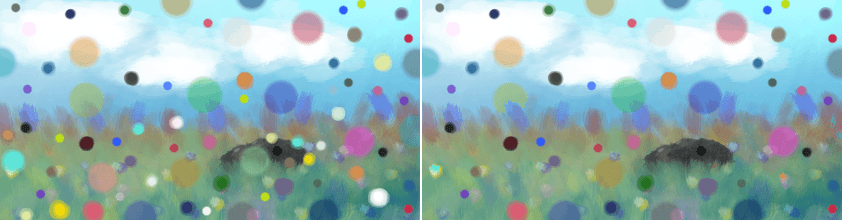Left: Normal. Right: Darker Color.

## Gamma Dark¶

Divides 1 by the upper layer, and calculates the end result using that as the power of the lower layer.

Darker Gray(0.4, 0.4, 0.4)^[1 / Lighter Gray(0.5, 0.5, 0.5)] = Even Darker Gray(0.1600, 0.1600, 0.1600)Left: Normal. Right: Gamma Dark.

Light Blue(0.1608, 0.6274, 0.8274)^[1 / Orange(1, 0.5961, 0.0706)] = Green(0.1608, 0.4575, 0.0683)Left: Normal. Right: Gamma Dark.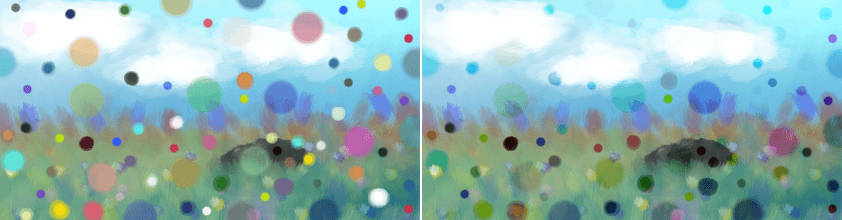Left: Normal. Right: Gamma Dark.

## Linear Burn¶

Adds the values of the two layers together and then subtracts 1. Seems to produce the same result as Inverse Subtract.

[Darker Gray(0.4, 0.4, 0.4) + Lighter Gray(0.5, 0.5, 0.5)]_1 = (-0.1000, -0.1000, -0.1000) → Black(0, 0, 0)Left: Normal. Right: Linear Burn.

[Light Blue(0.1608, 0.6274, 0.8274) + Orange(1, 0.5961, 0.0706)]_1 = (0.1608, 0.2235, -0.1020) → Dark Green(0.1608, 0.2235, 0)Left: Normal. Right: Linear Burn.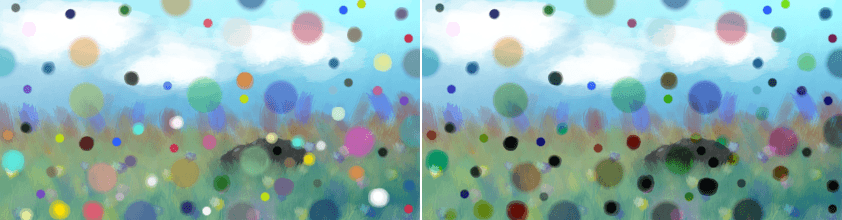Left: Normal. Right: Linear Burn.